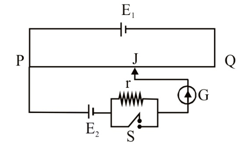A potentiometer wire PQ of length 1 m is connected to a standard cell E1. Another cell E2 of emf 1.02V is connected with a resistance ‘r’ and switch S (as shown in figure). With switch S open, the null position is obtained at a distance of 49cm from Q. The potential gradient in the potentiometer wire is:

# A potentiometer wire PQ of length 1 m is connected to a standard cell E1. Another cell E2 of emf 1.02V is connected with a resistance 'r' and switch S (as shown in figure). With switch S open, the null position is obtained at a distance of 49cm from Q. The potential gradient in the potentiometer wire is:1. A

$0.02\text{\hspace{0.17em}}\mathrm{V}/\mathrm{cm}$

2. B

$0.04\text{\hspace{0.17em}}\mathrm{V}/\mathrm{cm}$

3. C

$0.01\text{\hspace{0.17em}}\mathrm{V}/\mathrm{cm}$

4. D

$0.03\text{\hspace{0.17em}}\mathrm{V}/\mathrm{cm}$

Register to Get Free Mock Test and Study Material

+91

Verify OTP Code (required)

### Solution:

Balancing length is measured from P.
So $100-49=51\mathrm{cm}$
${\mathrm{E}}_{2}=\mathrm{\varphi }×51$
Where $\mathrm{\varphi }=$ Potential gradient
$\begin{array}{l}1.02=\mathrm{\varphi }×51\\ \mathrm{\varphi }=0.02\mathrm{V}/\mathrm{cm}\end{array}$Register to Get Free Mock Test and Study Material

+91

Verify OTP Code (required)